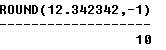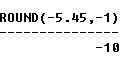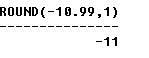## SQL ROUND (Number) Function

SQL ROUND (number) function is used to round numeric values. SQL ROUND function is similar to CEIL and FLOOR functions except that in this you mention the degree of precision you want to round upto. The syntax is:

ROUND (number, round_to);

Second parameter (round_to) defines the degree of precision you want in rounding this number. If the value is 2, it will round to 2 decimal places; if value is -1, it will round to 1 place to left of decimal. If you don’t pass any value for second parameter, it will round to zero decimal place.

Examples:
The following statements illustrate the use of SQL ROUND function on couple different numbers:

SELECT ROUND (2.32234234, 2) FROM DUAL;

OUTPUT:SELECT ROUND (12.342342, -1 ) FROM DUAL;

OUTPUT:SELECT ROUND (-5.45, -1) FROM DUAL;

OUTPUT:SELECT ROUND (-10.99, 1) FROM DUAL;

OUTPUT:Note: The sql round function uses the rule that if the digit under consideration is 4 or less, then it’s simply removed otherwise if the under consideration is 5 or more, then the number is rounded up to the next highest whole number and the digit is removed.